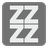Fizz Buzz
Elementary"Fizz buzz" is a word game we will use to teach the robots about division. Let's learn computers.

You should write a function that will receive a positive integer and return:
"Fizz Buzz" if the number is divisible by 3 and by 5;
"Fizz" if the number is divisible by 3;
"Buzz" if the number is divisible by 5;
The number as a string for other cases.

Input: A number as an integer.

Output: The answer as a string.

Example:

```checkio(15) == "Fizz Buzz"
checkio(6) == "Fizz"
checkio(5) == "Buzz"
checkio(7) == "7"

```

How it is used: Here you can learn how to write the simplest function and work with if-else statements.

Precondition: 0 < number ≤ 1000